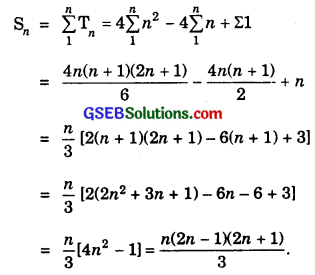# GSEB Solutions Class 11 Maths Chapter 9 Sequences and Series Ex 9.4

Gujarat Board GSEB Textbook Solutions Class 11 Maths Chapter 9 Sequences and Series Ex 9.4 Textbook Questions and Answers.

## Gujarat Board Textbook Solutions Class 11 Maths Chapter 9 Sequences and Series Ex 9.4

Find the sum of n terms of the following series:
1. 1 × 2 + 2 × 3 + 3 × 4 + 4 × 5 + ……….
2. 1 × 2 × 3 + 2 × 3 × 4 + 3 ×4 × 5 + ……….
3. 3 × 12 + 5 × 22 + 7 × 32 + ………
4. $$\frac{1}{1×2}$$ + $$\frac{1}{2×3}$$ + $$\frac{1}{3×4}$$ + …………….
5. 52 + 62 + 72 + ……………. + 202
6. 3 × 8 + 6 × 11 + 9 × 14 + ……………….
7. 12 + (12 + 22) + (12 + 22 + 32) + ………………..
Solutions to Q.No. 1 – 7:
1. Let S = 1 × 2 + 2 × 3 + 3 × 4 + 4 × 5 + …
now, nth term = n × (n + 1)
⇒ Tn = n2 + n.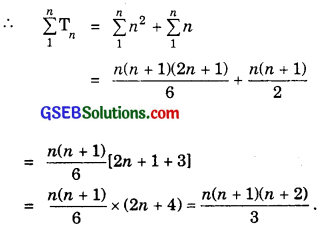2. Let Tn denote the nth term of the given series. Then,
Tn = [nth term of 1, 2, 3,…] [nth term of 2, 3, 4,…] [nth term of 3, 4, 5, …]
= [1 + (n – 1)1][2 + (n – 1). 1][3 + (n – 1).1]
= n(n + 1)(n + 2) = n(n2 + 2n + 3)
= n3 + 3n2 + 2n.
Sn = Σn3 + 3Σn2 + 2Σn.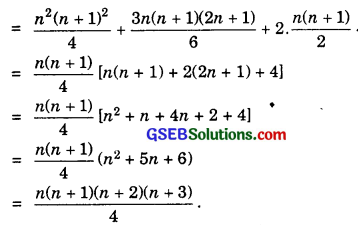3. Let Tn denote the nth term of the given series. Then,
Tn = [nth term of 3, 5, 7, …………..][nth term of 1, 2, 3, …………….]2
= [3 + 2(n – 1)23[1 + (n – 1).12] = (2n + 1)(n)
= n2(2n + 1) = 2n3 + n2.
So, Sn = 2Σn3 + Σn2.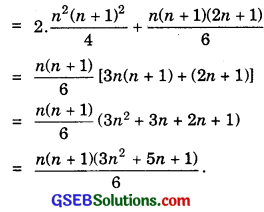4.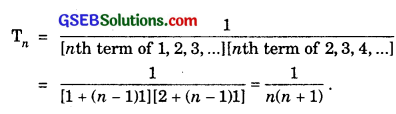Let $$\frac{1}{n(n+1)}$$ = $$\frac{A}{n}$$ + $$\frac{B}{n+1}$$ …………….. (1)
⇒ 1 = A(n + 1) + Bn …………………. (2) [On multiplying both sides by n(n + 1)]

To find B:
Put n = – 1 in (2), we get
1 = B(- 1) ⇒ B = – 1.
Put these values of A and B in (1), the partial fractions are
$$\frac{1}{n(n+1)}$$ = $$\frac{1}{n}$$ – $$\frac{1}{n+1}$$.
∴ Tn = $$\frac{1}{n}$$ – $$\frac{1}{n+1}$$.
Putting n = 1, 2, 3, ………….., n, we get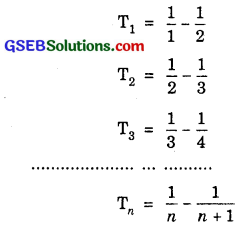Sn = 1 – $$\frac{1}{n+1}$$ = $$\frac{n+1-1}{n+1}$$ = $$\frac{n}{n+1}$$.

5. Tn of the given series
= (nth term of 5, 6, 7, ………….)2
= [5 + (n – 1).1]2
= (n + 4)2 = n2 + 8n + 16.
∴ Sn = Σn2 + 8Σn + 16 × n.
= $$\frac{n(n+1)(2n+1)}{6}$$ + 8 × $$\frac{n(n+1)}{2}$$ + 16n
= $$\frac{n}{6}$$[(n + 1)(2n + 1) + 24(n + 1) + 96]
= $$\frac{n}{6}$$[2n2 + n + 2n + 1 + 24n + 24 + 96]
= $$\frac{n}{6}$$(2n2 + 27n + 121).
Put n = 16, then
S = $$\frac{16}{6}$$ (2 × 162 + 27 × 16 + 121)
= $$\frac{8}{3}$$ (512 + 432 + 121) = $$\frac{8}{3}$$ × 1065
= 8 × 355 = 2840.6. Here, the series is formed by multiplying the corresponding terms of two series both of which are A.P.
viz., 3, 6, 9,… and 8, 11, 14, …
∴ Tn of given series = (nth term of 3, 6, 9, …) × (nth term of 8, 11, 14, …)
= [3 + (n – 1)3] [8 + (n – 1)3]
= [3n][3n + 5].
So, Sn = 9n2 + 15n = 9Σn2 + 15Σn.
= 9 × $$\frac{n(n+1)(2n+1)}{6}$$ + $$\frac{15.n(n+1)}{2}$$
= $$\frac{3}{2}$$.n(n + 1)[2n + 1 + 5].
= $$\frac{3}{2}$$.n(n + 1)(2n + 6) = 3n(n + 1)(n + 3).

7. Let Tn denote the nth term. Then,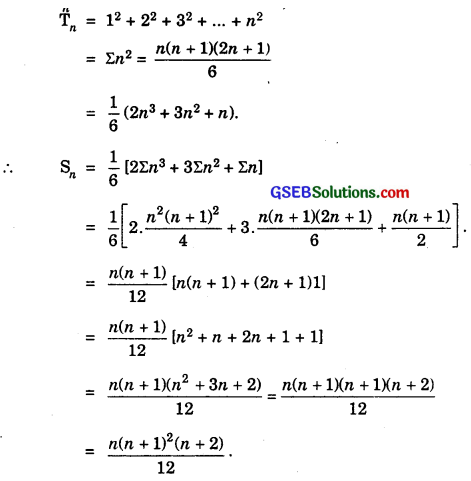Find the sum of first n terms of the following series whose nth terms are given by:
8. n(n + 1)(n + 4)
9. n2 + 2n
10. (2n – 1)2
Solutions to Q.No. 8 – 10:
8. Tn = n(n + 1)(n + 4) = n(n2 + 5n + 4)
= n3 + 5n2 + 4n.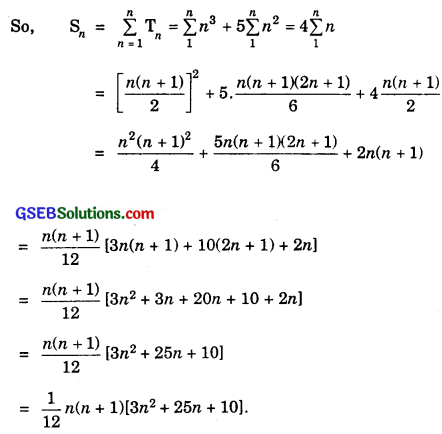9. Tn = n2 + 2n
Put n = 1, 2, 3, ………….. n, we get
T1 = 12 + 21
T2 = 22 + 22
T3 = 32 + 23
……………………
Tn = n2 + 2n
= Σn2 + $$\frac{2\left(2^{n}-1\right)}{2-1}$$ = $$\frac{n(n + 1)(2n + 1)}{6}$$ + 2(2n – 1).